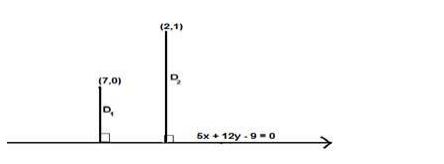# Show that the length of the perpendicular from the pointQuestion:

Show that the length of the perpendicular from the point (7, 0) to the line 5x + 12y – 9 = 0 is double the length of perpendicular to it from the point (2, 1)

Solution:

Given: Points (7,0) and (2,1) , line 5x + 12y – 9 = 0

To Prove : length of the perpendicular from the point $(7,0)$ to the line $5 x+12 y-9=0$ is double the length of perpendicular to it from the point $(2,1)$

Formula used: We know that the length of the perpendicular from $(\mathrm{m}, \mathrm{n})$ to the line $\mathrm{ax}+$ by $+c=0$ is given by,

$D=\frac{|a m+b n+c|}{\sqrt{a^{2}+b^{2}}}$Let $D_{1}$ be the length of perpendicular from the point $(7,0)$ to the line $5 x+12 y-9=0$

The given equation of the line is $5 x+12 y-9=0$

Here at point $(7,0) \mathrm{m}=7$ and $\mathrm{n}=0, \mathrm{a}=5, \mathrm{~b}=12, \mathrm{c}=-9$

$D_{1}=\frac{|5(7)+12(0)-9|}{\sqrt{5^{2}+12^{2}}}$

$D_{1}=\frac{|35+0-9|}{\sqrt{25+144}}=\frac{26}{\sqrt{169}}=\frac{26}{13}=2$

$D_{1}=2$

Let $D_{2}$ be the length of perpendicular from the point $(2,1)$ to the line $5 x+12 y-9=0$

The given equation of the line is $5 x+12 y-9=0$

Here at point $(2,1) m=2$ and $n=1, a=5, b=12, c=-9$

$D_{2}=\frac{|5(2)+12(1)-9|}{\sqrt{5^{2}+12^{2}}}$

$D_{2}=\frac{|10+12-9|}{\sqrt{25+144}}=\frac{22-9}{\sqrt{169}}=\frac{13}{13}=1$

$D_{2}=1$

$D_{1}=2 D_{2}=2$

Thus the length of the perpendicular from the point $(7,0)$ to the line $5 x+12 y-9=0$ is double the length of perpendicular to it from the point $(2,1)$